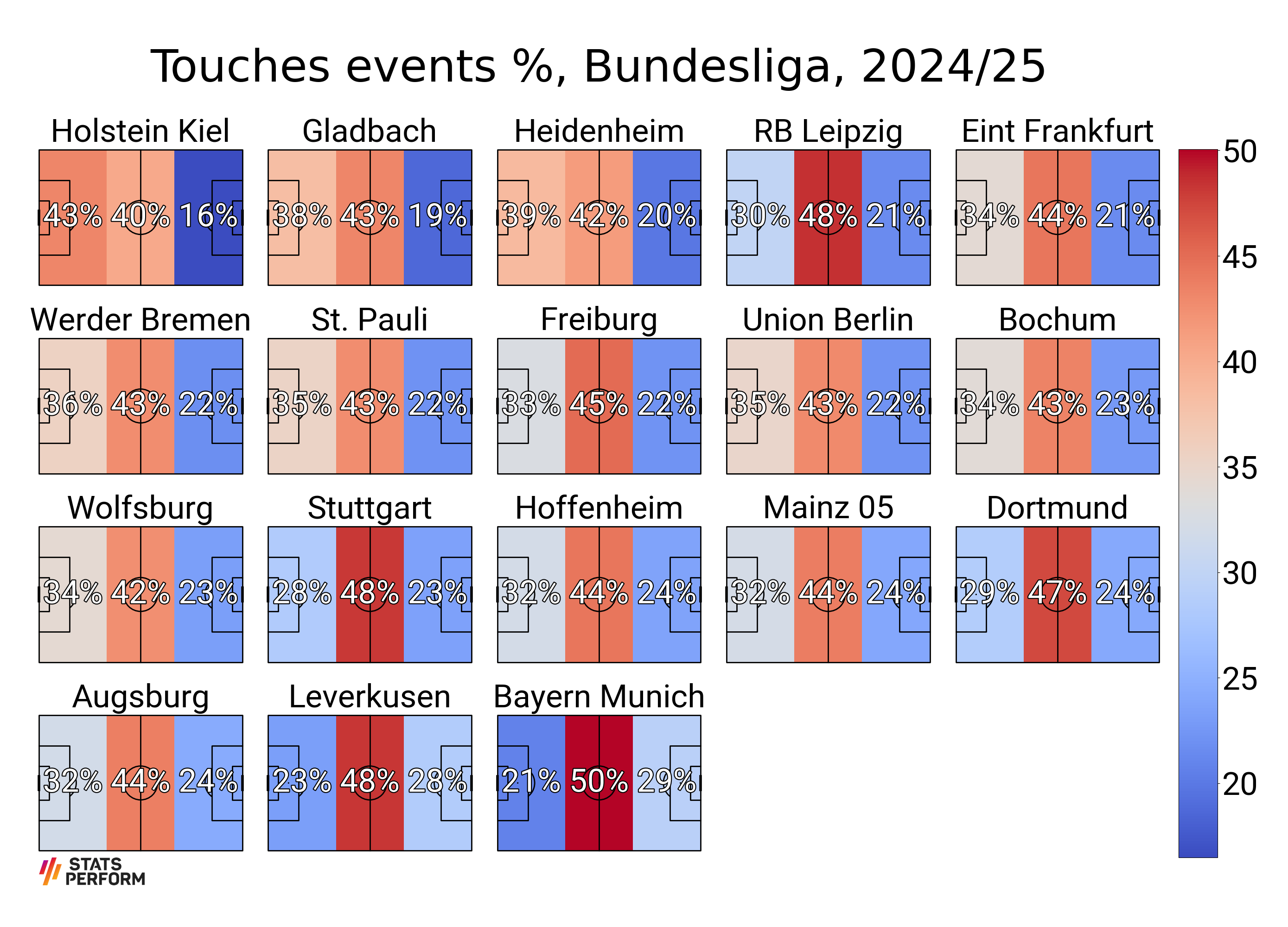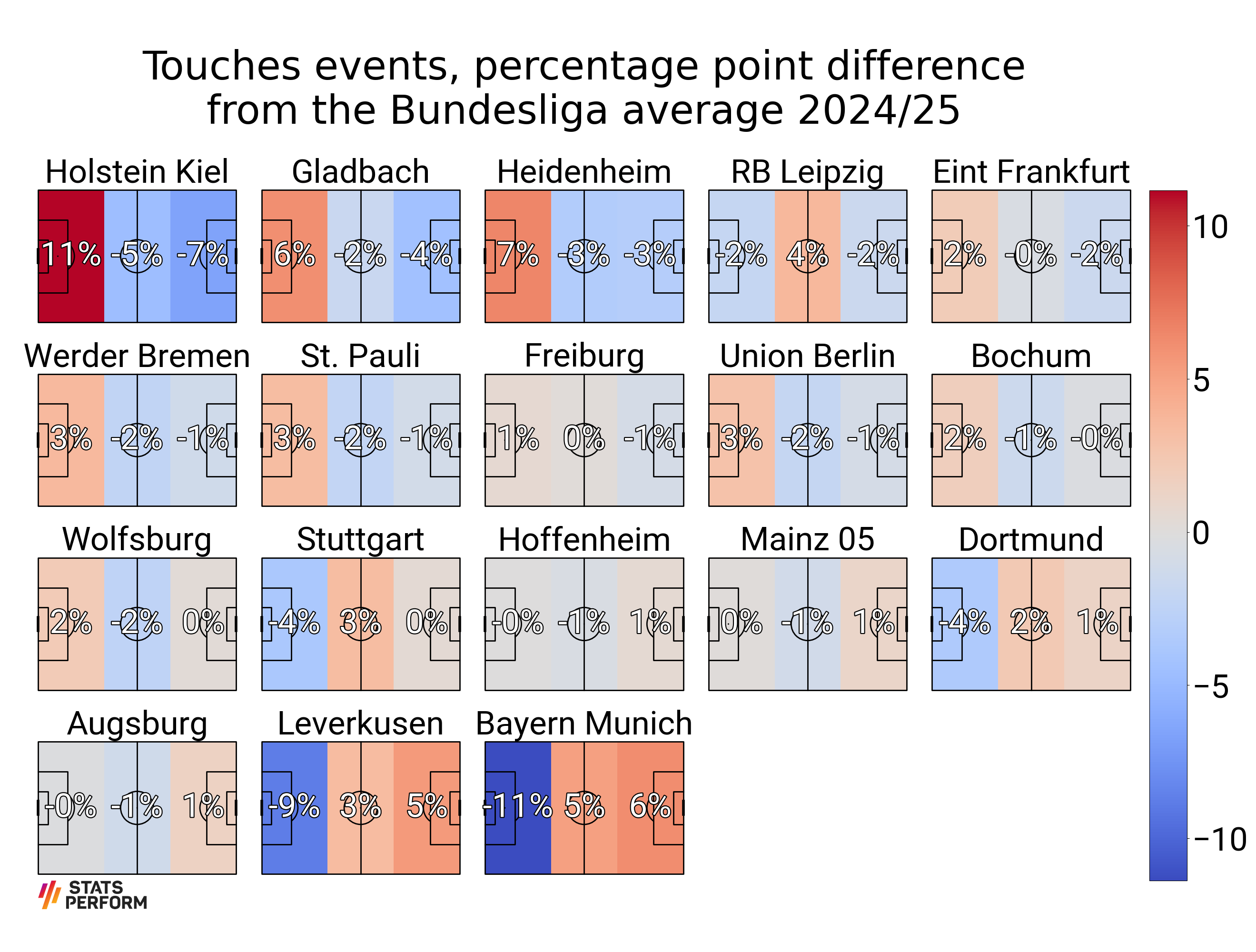# FBRef Touches

This example shows how to scrape touches events from FBRef.com and plot them as a heatmap.

```from urllib.request import urlopen

import matplotlib.patheffects as path_effects
import matplotlib.pyplot as plt
import pandas as pd
from PIL import Image

from mplsoccer import Pitch, FontManager, add_image
```

Scrape the data via a link to a specific table. To get the link for a different league, find the table you want from the website. Then click “Share & more” and copy the link from the option “Modify & Share Table”. Then “click url for sharing” and get the table as a url.

```URL = 'https://fbref.com/en/share/LdLSY'
# select a subset of the columns (Squad and touches columns)
df = df[['Unnamed: 0_level_0', 'Touches']].copy()
df.columns = df.columns.droplevel()  # drop the top-level of the multi-index
df = df.drop(["Def Pen", "Att Pen", "Live"], axis = 1) # drop the def pen, att pen, live touches column
```

Get the league average percentages

```touches_cols = ['Def 3rd', 'Mid 3rd', 'Att 3rd']
df_total = pd.DataFrame(df[touches_cols].sum())
df_total.columns = ['total']
df_total = df_total.T
df_total = df_total.divide(df_total.sum(axis=1), axis=0) * 100
```

Calculate the percentages for each team and sort so that the teams make the most touches are last

```df[touches_cols] = df[touches_cols].divide(df[touches_cols].sum(axis=1), axis=0) * 100.
df.sort_values(['Att 3rd', 'Def 3rd'], ascending=[True, False], inplace=True)
```

Get Stats Perform’s logo and Fonts

```SP_LOGO_URL = ('https://upload.wikimedia.org/wikipedia/commons/d/d5/StatsPerform_Logo_Primary_01.png')
sp_logo = Image.open(urlopen(SP_LOGO_URL))

# a FontManager object for using a google font (default Robotto)
fm = FontManager()
# path effects
path_eff = [path_effects.Stroke(linewidth=3, foreground='black'),
path_effects.Normal()]
```

Plot the percentages

```# setup a mplsoccer pitch

# mplsoccer calculates the binned statistics usually from raw locations, such as touches events
# for this example we will create a binned statistic dividing
# the pitch into thirds for one point (0, 0)
# we will fill this in a loop later with each team's statistics from the dataframe
bin_statistic = pitch.bin_statistic(, , statistic='count', bins=(3, 1))

GRID_HEIGHT = 0.8
CBAR_WIDTH = 0.03
fig, axs = pitch.grid(nrows=4, ncols=5, figheight=20,
# leaves some space on the right hand side for the colorbar
grid_width=0.88, left=0.025,
endnote_height=0.03, endnote_space=0,
# Turn off the endnote/title axis. I usually do this after
# I am happy with the chart layout and text placement
axis=False,
title_space=0.02, title_height=0.06, grid_height=GRID_HEIGHT)
fig.set_facecolor('white')

vmin = df[touches_cols].min().min()  # we normalise the heatmaps with the min / max values
vmax = df[touches_cols].max().max()
for i, ax in enumerate(axs['pitch'].flat[:len(teams)]):
# the top of the pitch is zero
# plot the title half way between zero and -20 (the top padding)
ax.text(60, -10, teams[i],
ha='center', va='center', fontsize=50,
fontproperties=fm.prop)

# fill in the bin statistics from df and plot the heatmap
bin_statistic['statistic'] = df.loc[df.Squad == teams[i], touches_cols].values
heatmap = pitch.heatmap(bin_statistic, ax=ax, cmap='coolwarm', vmin=vmin, vmax=vmax)
annotate = pitch.label_heatmap(bin_statistic, color='white', fontproperties=fm.prop,
path_effects=path_eff, fontsize=50, ax=ax,
str_format='{0:.0f}%', ha='center', va='center')

# if its the Bundesliga remove the two spare pitches
if len(teams) == 18:
for ax in axs['pitch'][-1, 3:]:
ax.remove()

cbar_bottom = axs['pitch'][-1, 0].get_position().y0
cbar_left = axs['pitch'][0, -1].get_position().x1 + 0.01
# take a little bit off the height because of padding
GRID_HEIGHT - 0.036))
cbar = plt.colorbar(heatmap, cax=ax_cbar)
for label in cbar.ax.get_yticklabels():
label.set_fontproperties(fm.prop)
label.set_fontsize(50)

# title and endnote
left=axs['endnote'].get_position().x0,
bottom=axs['endnote'].get_position().y0,
height=axs['endnote'].get_position().height)
title = axs['title'].text(0.5, 0.5, 'Touches events %, Bundesliga, 2022/23',
ha='center', va='center', fontsize=70)
```Plot the percentage point difference

```# Calculate the percentage point difference from the league average
df[touches_cols] = df[touches_cols].values - df_total.values

GRID_HEIGHT = 0.76
fig, axs = pitch.grid(nrows=4, ncols=5, figheight=20,
# leaves some space on the right hand side for the colorbar
grid_width=0.88, left=0.025,
endnote_height=0.03, endnote_space=0,
# Turn off the endnote/title axis. I usually do this after
# I am happy with the chart layout and text placement
axis=False,
title_space=0.02, title_height=0.1, grid_height=GRID_HEIGHT)
fig.set_facecolor('white')

vmin = df[touches_cols].min().min()  # we normalise the heatmaps with the min / max values
vmax = df[touches_cols].max().max()

for i, ax in enumerate(axs['pitch'].flat[:len(teams)]):
# the top of the pitch is zero
# plot the title half way between zero and -20 (the top padding)
ax.text(60, -10, teams[i], ha='center', va='center', fontsize=50, fontproperties=fm.prop)

# fill in the bin statistics from df and plot the heatmap
bin_statistic['statistic'] = df.loc[df.Squad == teams[i], touches_cols].values
heatmap = pitch.heatmap(bin_statistic, ax=ax, cmap='coolwarm', vmin=vmin, vmax=vmax)
annotate = pitch.label_heatmap(bin_statistic, color='white', fontproperties=fm.prop,
path_effects=path_eff, str_format='{0:.0f}%', fontsize=50,
ax=ax, ha='center', va='center')

# if its the Bundesliga remove the two spare pitches
if len(teams) == 18:
for ax in axs['pitch'][-1, 3:]:
ax.remove()

cbar_bottom = axs['pitch'][-1, 0].get_position().y0
cbar_left = axs['pitch'][0, -1].get_position().x1 + 0.01
# take a little bit off the height because of padding
GRID_HEIGHT - 0.035))
cbar = plt.colorbar(heatmap, cax=ax_cbar)
for label in cbar.ax.get_yticklabels():
label.set_fontproperties(fm.prop)
label.set_fontsize(50)

# title and endnote